## Length Mass Volume: Real Life 1-Step Word Problems

Practice Unlimited Questions

#### 1. A dictionary has a mass of 1478 g. A diary has a mass of 1872 g. What is their total mass in kilograms and grams?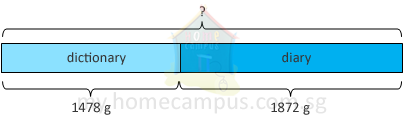1478 g  +  1872 g
=  3350 g
=  3000 g  +  350 g
=  3 kg  +  350 g
=  3 kg 350 g

Their total mass is 3 kg 350 g.

#### 2. Chelsea's house is 8 km from the theme park. It is 5 times as far from the water park as the theme park. Find the distance between Chelsea's house and the water park.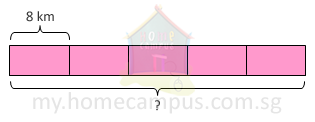8 km   ×   5   =   40 km

The distance between Chelsea's house and the water park is 40 km.

#### 3. A sack of wheat has a mass of 31 kg. A sack of corn has a mass of 18 kg. How much heavier is the sack of wheat than the sack of corn?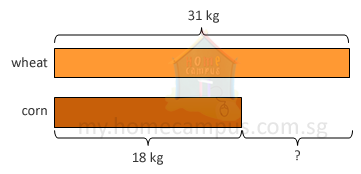31 kg   −   18 kg   =   13 kg

The sack of wheat is 13 kg heavier than the sack of corn.

#### 4. Olivia has a plastic wrap of length 305 cm. She cuts it into 5 pieces. What is the length of each piece?305 cm   ÷   5   =   61 cm

The length of each piece is 61 cm.

#### 5. Jeff had 218 ml of glycerine. He had 71 ml less glycerine than Mia. How much glycerine had Mia?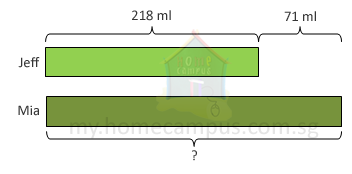218 ml  +  71 ml  =  289 ml

Mia had 289 ml of glycerine.

#### 6. Taylor had 1000 g of brown rice. He packed the brown rice into bags of 200 g each. How many bags did he make?1000 g  ÷  200 g  =  5

1000 ÷ 200 can be thought of as 10 ÷ 2.

#### 7. Halle bought 4 m of gift wrapper. She used 165 cm of it to wrap a gift. What is the length of gift wrapper she had left?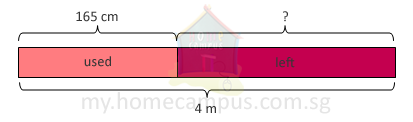4 m  −  165 cm
=  400 cm  −  165 cm
=  235 cm
=  200 cm  +  35 cm
=  2 m  +  35 cm
=  2 m 35 cm